# Math 753/853 HW2¶

Problem 1. Write a Julia function bisectsearch that takes a function $f(x)$ and an interval $a,b$ such that $f(a) f(b) < 0$, returns a root $r$ of $f$ within that interval. Find the root to as many digits accuracy as possible.

Challenges:

• Add checks to your bisectsearch function that verify that the starting conditions for bisection search are met. The checks should print a helpful error message and exit the function while returning the most reasonable value for the root from available information.

• Think carefully about the stopping conditions for bisection search. What kinds of problems are possible for this algorithm when it's implemented in floating-point arthmetic? Add checks to your function that stop iteration and return the most reasonable value for the root. In some cases you might want to print an error or warning message.

• Add some diagnostic printing to your function that prints out $a,b$, and $f(c)$ at each iteration. Add another argument diagnostics to the function that turns the printing on and off. Make diagnostics default to false.

• Write your bisectsearch function so that it operates equally well on any floating-point type: Float16, Float32, Float64, or BigFloat.

• Instead of using if a; b; end statements for your checks, use the more Juliaesque a && b short-circuit conditional evaluation. Likewise, instead of using if a; b ; else c ; end statements, use the a ? b : c ternary operator. See the Julia docs on conditional expressions.

In :
function bisectsearch(f, a, b, diagnostics=false)

c = float((a+b)/2)
ftolerance = 10*eps(typeof(c))       # set tolerance = 10 machine eps
nmax = 1000;                         # some large but finite number

(fa, fb)  = (f(a), f(b))

if fa*fb > zero(c)
println("bisectsearch(f,a,b) error: a,b do not bracket a zero")
return c
end

n = 0  # counter for iterations
while abs(b-a) > eps(c) && n < nmax

c = float((a+b)/2)
fc = f(c)

# example of compact short-circuit conditional evaluation syntax
# in place of bulkier if-end
diagnostics && println("n == $n, a,b ==$a, $b, f(c) ==$fc")

# same as if  abs(fc) < ftolerance; return c; end
abs(fc) < ftolerance && return c

# replace either (a, fa) or (b, fb) with (c, fc)
# using ternary x ? y : z operator to
sign(fc) == sign(fa) ? (a,fa) = (c,fc) : (b,fb) = (c,fc)

n += 1 # increment iteration counter
end

# another short-circuit conditional evaluation
diagnostics && println("bisectsearch(f,a,b) warning: exiting without convergence")

end

Out:
bisectsearch (generic function with 2 methods)

Problem 2. Apply the bisection search algorithm to find all real roots of

(a) $3x^3 + x^2 = x + 5$

In :
f(x) = 3x.^3 + x.^2 - x - 5
using PyPlot
x = linspace(-2, 2);
plot(x, f(x))
xlabel("x"), ylabel("f(x) = 3x^3 + x^2 - x - 5"); grid("on")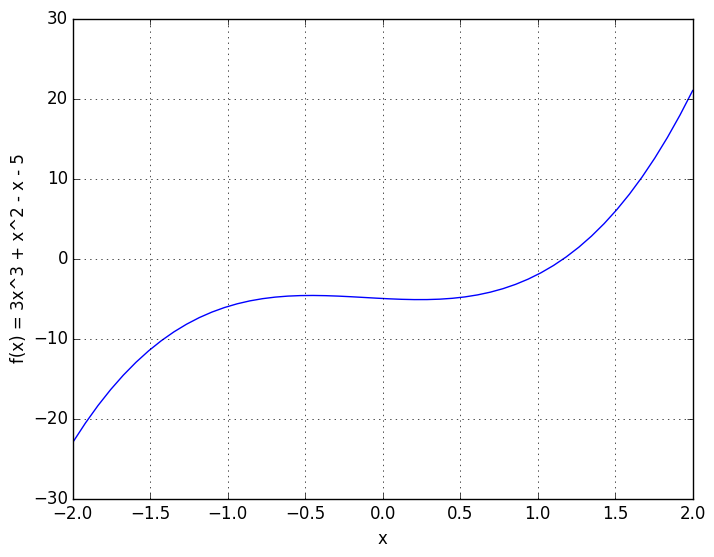In :
# Looks like it f(x) = 3x^3 + x^2 - x - 5 has a single root near x=1. Bracketed by [a,b] = [0, 2].
r = bisectsearch(f, 0, 2)

Out:
1.1697262198537244
In :
# Evaluate f(r), make sure it is very small
f(r)

Out:
8.881784197001252e-16

(b) $\cos^2 x + 6 = x$

In :
# give this function a different name so we can reuse it in the Newton search problem
g(x) = cos(x).^2 + 6 - x
x = linspace(0, 10);
plot(x, g(x))
xlabel("x"), ylabel("g(x) = cos^2 x - x + 6"); grid("on")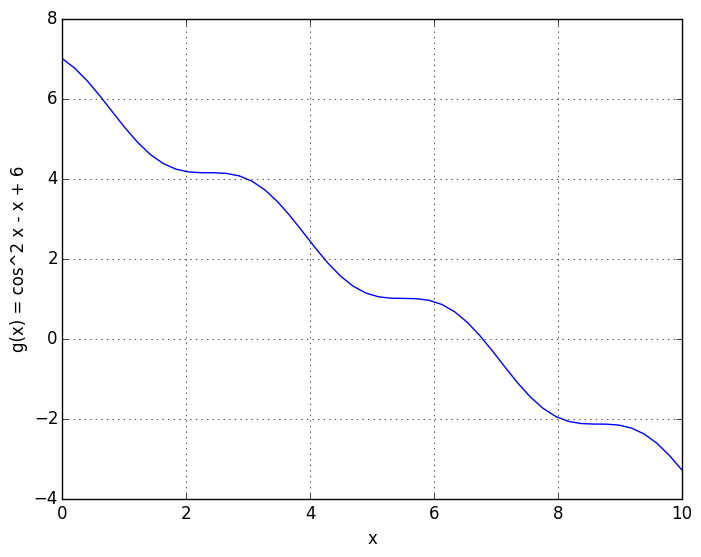In :
# one root bracketed by [6,8]
r = bisectsearch(g, 6, 8)

Out:
6.776092316319502
In :
g(r)

Out:
0.0

(c) $\ln x + x^2 = 3$

In :
h(x) = log(x) + x.^2 - 3
x = linspace(0.001, 4)
plot(x, h(x))
xlabel("x"), ylabel("h(x) = cos^2 x - x + 6"); grid("on")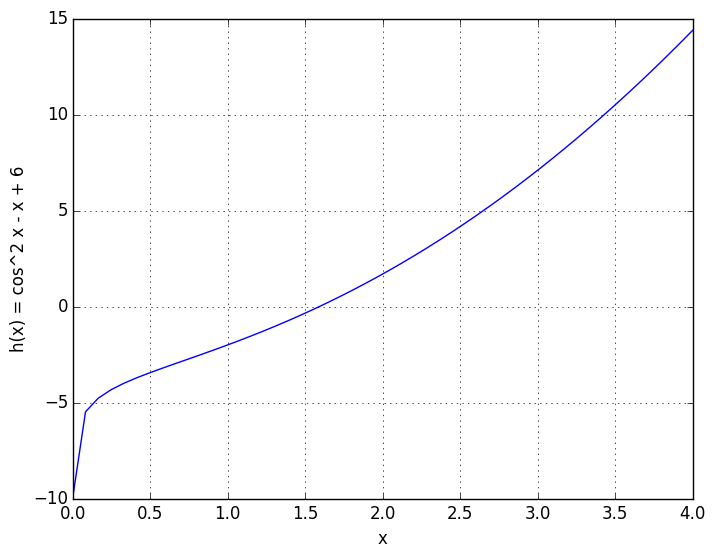In :
r = bisectsearch(h,1,2)

Out:
1.5921429370580942
In :
h(r)

Out:
8.881784197001252e-16
In :
r = bisectsearch(f,1,2,true)

n == 0,  a,b == 1, 2,  f(c) ==5.875
n == 1,  a,b == 1, 1.5,  f(c) ==1.171875
n == 2,  a,b == 1, 1.25,  f(c) ==-0.587890625
n == 3,  a,b == 1.125, 1.25,  f(c) ==0.246337890625
n == 4,  a,b == 1.125, 1.1875,  f(c) ==-0.181915283203125
n == 5,  a,b == 1.15625, 1.1875,  f(c) ==0.029392242431640625
n == 6,  a,b == 1.15625, 1.171875,  f(c) ==-0.07696199417114258
n == 7,  a,b == 1.1640625, 1.171875,  f(c) ==-0.023960530757904053
n == 8,  a,b == 1.16796875, 1.171875,  f(c) ==0.0026718750596046448
n == 9,  a,b == 1.16796875, 1.169921875,  f(c) ==-0.010655314661562443
n == 10,  a,b == 1.1689453125, 1.169921875,  f(c) ==-0.00399446755181998
n == 11,  a,b == 1.16943359375, 1.169921875,  f(c) ==-0.000661983314785175
n == 12,  a,b == 1.169677734375, 1.169921875,  f(c) ==0.0010047740888694534
n == 13,  a,b == 1.169677734375, 1.1697998046875,  f(c) ==0.0001713524432034319
n == 14,  a,b == 1.169677734375, 1.16973876953125,  f(c) ==-0.000245326171494753
n == 15,  a,b == 1.169708251953125, 1.16973876953125,  f(c) ==-3.698954810360533e-5
n == 16,  a,b == 1.1697235107421875, 1.16973876953125,  f(c) ==6.71807765559862e-5
n == 17,  a,b == 1.1697235107421875, 1.1697311401367188,  f(c) ==1.5095446478596841e-5
n == 18,  a,b == 1.1697235107421875, 1.1697273254394531,  f(c) ==-1.0947092749624687e-5
n == 19,  a,b == 1.1697254180908203, 1.1697273254394531,  f(c) ==2.0741663799839216e-6
n == 20,  a,b == 1.1697254180908203, 1.1697263717651367,  f(c) ==-4.4364658062789886e-6
n == 21,  a,b == 1.1697258949279785, 1.1697263717651367,  f(c) ==-1.1811503686232072e-6
n == 22,  a,b == 1.1697261333465576, 1.1697263717651367,  f(c) ==4.46507842255528e-7
n == 23,  a,b == 1.1697261333465576, 1.1697262525558472,  f(c) ==-3.673213040400469e-7
n == 24,  a,b == 1.1697261929512024, 1.1697262525558472,  f(c) ==3.959325933777791e-8
n == 25,  a,b == 1.1697261929512024, 1.1697262227535248,  f(c) ==-1.6386402457158056e-7
n == 26,  a,b == 1.1697262078523636, 1.1697262227535248,  f(c) ==-6.213538306099053e-8
n == 27,  a,b == 1.1697262153029442, 1.1697262227535248,  f(c) ==-1.1271063193873943e-8
n == 28,  a,b == 1.1697262190282345, 1.1697262227535248,  f(c) ==1.4161098960130403e-8
n == 29,  a,b == 1.1697262190282345, 1.1697262208908796,  f(c) ==1.44501832721744e-9
n == 30,  a,b == 1.1697262190282345, 1.169726219959557,  f(c) ==-4.913022877417461e-9
n == 31,  a,b == 1.1697262194938958, 1.169726219959557,  f(c) ==-1.7340031632784303e-9
n == 32,  a,b == 1.1697262197267264, 1.169726219959557,  f(c) ==-1.4449152985207547e-10
n == 33,  a,b == 1.1697262198431417, 1.169726219959557,  f(c) ==6.502620664150527e-10
n == 34,  a,b == 1.1697262198431417, 1.1697262199013494,  f(c) ==2.5288571237069846e-10
n == 35,  a,b == 1.1697262198431417, 1.1697262198722456,  f(c) ==5.419664717010164e-11
n == 36,  a,b == 1.1697262198431417, 1.1697262198576936,  f(c) ==-4.5147885430196766e-11
n == 37,  a,b == 1.1697262198504177, 1.1697262198576936,  f(c) ==4.524380869952438e-12
n == 38,  a,b == 1.1697262198504177, 1.1697262198540557,  f(c) ==-2.0311752280122164e-11
n == 39,  a,b == 1.1697262198522367, 1.1697262198540557,  f(c) ==-7.893241615875013e-12
n == 40,  a,b == 1.1697262198531462, 1.1697262198540557,  f(c) ==-1.6830981053317373e-12
n == 41,  a,b == 1.169726219853601, 1.1697262198540557,  f(c) ==1.4193091146808001e-12
n == 42,  a,b == 1.169726219853601, 1.1697262198538283,  f(c) ==-1.3322676295501878e-13
n == 43,  a,b == 1.1697262198537146, 1.1697262198538283,  f(c) ==6.439293542825908e-13
n == 44,  a,b == 1.1697262198537146, 1.1697262198537715,  f(c) ==2.5579538487363607e-13
n == 45,  a,b == 1.1697262198537146, 1.169726219853743,  f(c) ==6.217248937900877e-14
n == 46,  a,b == 1.1697262198537146, 1.1697262198537288,  f(c) ==-3.4638958368304884e-14
n == 47,  a,b == 1.1697262198537217, 1.1697262198537288,  f(c) ==1.3322676295501878e-14
n == 48,  a,b == 1.1697262198537217, 1.1697262198537253,  f(c) ==-1.0658141036401503e-14
n == 49,  a,b == 1.1697262198537235, 1.1697262198537253,  f(c) ==8.881784197001252e-16

Out:
1.1697262198537244
In :
# Let's also test it on 32-bit floats
r = bisectsearch(h,1.0f0, 2.0f0,true)

n == 0,  a,b == 1.0, 2.0,  f(c) ==-0.34453487
n == 1,  a,b == 1.5, 2.0,  f(c) ==0.62211585
n == 2,  a,b == 1.5, 1.75,  f(c) ==0.12613273
n == 3,  a,b == 1.5, 1.625,  f(c) ==-0.112306595
n == 4,  a,b == 1.5625, 1.625,  f(c) ==0.006128788
n == 5,  a,b == 1.5625, 1.59375,  f(c) ==-0.05328417
n == 6,  a,b == 1.578125, 1.59375,  f(c) ==-0.023626566
n == 7,  a,b == 1.5859375, 1.59375,  f(c) ==-0.0087611675
n == 8,  a,b == 1.5898438, 1.59375,  f(c) ==-0.00131917
n == 9,  a,b == 1.5917969, 1.59375,  f(c) ==0.0024039745
n == 10,  a,b == 1.5917969, 1.5927734,  f(c) ==0.00054216385
n == 11,  a,b == 1.5917969, 1.5922852,  f(c) ==-0.00038862228
n == 12,  a,b == 1.592041, 1.5922852,  f(c) ==7.70092f-5
n == 13,  a,b == 1.592041, 1.5921631,  f(c) ==-0.00015592575
n == 14,  a,b == 1.592102, 1.5921631,  f(c) ==-3.9577484f-5
n == 15,  a,b == 1.5921326, 1.5921631,  f(c) ==1.859665f-5
n == 16,  a,b == 1.5921326, 1.5921478,  f(c) ==-1.04904175f-5
n == 17,  a,b == 1.5921402, 1.5921478,  f(c) ==4.053116f-6
n == 18,  a,b == 1.5921402, 1.592144,  f(c) ==-3.33786f-6
n == 19,  a,b == 1.5921421, 1.592144,  f(c) ==4.7683716f-7

Out:
1.592143f0
In :
h(r)

Out:
4.7683716f-7

Problem 3. Write a Julia function newtonsearch that take a function $f(x)$ and an initial guess $x_0$, and returns a root $r$ of $f$ using the Newton method. Find the root to as many digits accuracy as possible.

Challenges:

• Unlike bisection, which is guaranteed to converge, the Newton method can go haywire. Think of a good way to test if the Newton method is failing, and print an error message and exit in this case. Your function should still return a number of the same floating-point type as $x_0$. In Julia, this is known as type stability.
• as in the bisection search, think carefully about the appropriate stopping conditions for the search, given the finite precision of computer arithmetic
• make sure your code operates equally well on all floating-point types.
• add diagnostic printing to your code and turn it on/off with a diagnostics argument that defaults to false
• use short-circuit boolean and ternary operators instead of if-then, where appropriate, to make your code more compact and readable
In :
function newtonsearch(f, x, diagnostics=false)

x = float(x)
fx = f(x)
ftolerance = 10*eps(typeof(float(fx)))

n=0;       # iteration counter
nmax=100;  # max iterations

while abs(fx) > ftolerance && n<100;

diagnostics && println("n=$n, x=$x, f(x)=$fx"); # determine a small dx, relative to x, but larger than eps(x) dx = sqrt(eps(x)); # compute Newton step fprime = (f(x + dx) - fx)/dx x = x - fx/fprime # update fx and n fx = f(x) n = n+1 end x end  Out: newtonsearch (generic function with 2 methods) Problem 4. Apply the Newton method to find all real roots of (a)$3x^3 + x^2 = x + 5$In : r = newtonsearch(f,1)  Out: 1.1697262198537244 In : f(r)  Out: 8.881784197001252e-16 (b)$\cos^2 x + 6 = x$In : r = newtonsearch(g,7)  Out: 6.776092316319502 In : g(r)  Out: 0.0 (c)$\ln x + x^2 = 3$In : p(x) = log(x) + x^2 - 3  Out: p (generic function with 1 method) In : # Let's try this one in high precision, using BigFloat r = newtonsearch(h, BigFloat(1))  Out: 1.592142937058093867781640717162575608487041280209297260897580871974043026175967 In : h(r)  Out: 0.000000000000000000000000000000000000000000000000000000000000000000000000000000 In : # Let's check out the converegence, step-by-step, using the diagnostic print statements r = newtonsearch(h, 1.0, true)  n=0, x=1.0, f(x)=-2.0 n=1, x=1.6666666633552976, f(x)=0.28860338851905 n=2, x=1.593292920585597, f(x)=0.004385224652566855 n=3, x=1.5921432154543025, f(x)=1.0613494678679558e-6 n=4, x=1.592142937058112, f(x)=6.927791673660977e-14  Out: 1.5921429370580937 Problem 5. Modify your bisection-search and Newton-method functions so that, along with the root$r$they return a vector of errors$e_n = | x_n - r|$for$n=1,2,...$. Then solve$\cos^2 x + 6 = x$using both bisection and Newton method, and make a plot comparing$e_n$versus$n$for the two methods. Put both the bisection errors and the Newton method errors on the same plot. Use blue circles for bisection errors and red squares for Newton method. Make the vertical$e_n$axis logarithmic. In : function bisectsearch(f, a, b, diagnostics=false) c = float((a+b)/2) ftolerance = 10*eps(typeof(c)) # set tolerance = 10 machine eps nmax = 1000; # some large but finite number (fa, fb) = (f(a), f(b)) if fa*fb > zero(c) println("bisectsearch(f,a,b) error: a,b do not bracket a zero") return c end n = 1 # counter for iterations err = [] # vector for returning errors at each stage while abs(b-a) > eps(c) && n < nmax c = float((a+b)/2) fc = f(c) push!(err,c) # append current value of c to error vector # example of compact short-circuit conditional evaluation syntax # in place of bulkier if-end diagnostics && println("n ==$n,  a,b == $a,$b,  f(c) ==$fc") # same as if abs(fc) < ftolerance; break; end abs(fc) < ftolerance && break # replace either (a, fa) or (b, fb) with (c, fc) # using ternary x ? y : z operator to sign(fc) == sign(fa) ? (a,fa) = (c,fc) : (b,fb) = (c,fc) n += 1 # increment iteration counter end pop!(err) # take last value of c off the err vector err = abs(err-c) # convert values of c to errors (c,err) # return c and vector of errors end function newtonsearch(f, x, diagnostics=false) x = float(x) fx = f(x) ftolerance = 10*eps(typeof(float(fx))) n=0 # iteration counter nmax=100 # max iterations err = [] # vector for string errors, step by step while abs(fx) > ftolerance && n<100; diagnostics && println("n=$n, x=$x, f(x)=$fx");

# determine a small dx, relative to x, but larger than eps(x)
dx = sqrt(eps(x))

# compute Newton step
fprime = (f(x + dx) - fx)/dx
x = x - fx/fprime

# update fx, n, and error
fx = f(x)
n += 1
push!(err, x)

end
pop!(err)
err = abs(err-x)
(x,err)
end

WARNING: Method definition bisectsearch(Any, Any, Any) in module Main at In:3 overwritten at In:3.
WARNING: Method definition bisectsearch(Any, Any, Any, Any) in module Main at In:3 overwritten at In:3.
WARNING: Method definition newtonsearch(Any, Any) in module Main at In:3 overwritten at In:44.
WARNING: Method definition newtonsearch(Any, Any, Any) in module Main at In:3 overwritten at In:44.

Out:
newtonsearch (generic function with 2 methods)
In [ ]:



We have theoretical estimates of the convergence rates of bisection and Newton method. Do your error plots fit this theory, or not? Explain.

In :
(rbisect, errbisect) = bisectsearch(g,6,8)

Out:
(6.776092316319502,[0.223908,0.276092,0.0260923,0.0989077,0.0364077,0.00515768,0.0104673,0.00265482,0.00125143,0.000701691  …  8.20677e-13,9.98313e-13,8.88178e-14,3.6593e-13,1.38556e-13,2.4869e-14,3.19744e-14,3.55271e-15,1.06581e-14,3.55271e-15])
In :
(rnewton, errnewton) = newtonsearch(g,7)

Out:
(6.776092316319502,[0.00707367,1.47899e-5,6.60254e-11])
In :
semilogy(errnewton, "ro")
semilogy(errbisect, "bo")
n = 1:45
semilogy(n, 0.5.^n, "k--")
xlabel("iteration number n")
ylabel("error |x_n - r|")
legend(("newton search", "bisect search", "2^-n"))
title("convergence of newton and bisection search on g(x) = cos(x)^2 + 6 - x")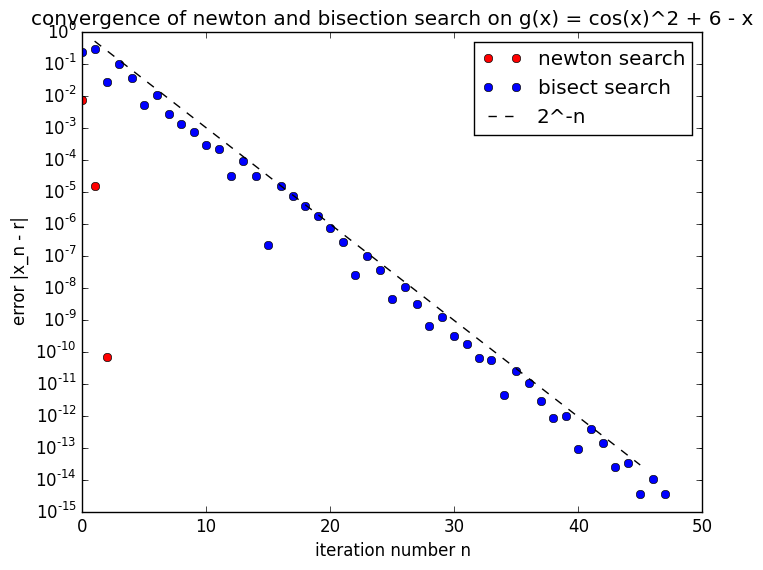Out:
PyObject <matplotlib.text.Text object at 0x7f002b29c290>

We have theoretical estimates of the convergence rates of bisection and Newton method. Do your error plots fit this theory, or not? Explain.

In :
# Yes, the curves fit the estimates for both Newton and bisection.
# For Newton, the number of digits of precision approximately doubles every iteration: 1e-2, 1e-5, 1e-10
# For bisection, the error scales as 2^-n as expected


## Bonus problems!¶

Bonus problems are extra challenges for those who just can't get enough numerical mathematics!

Bonus problem 6. Use your Newton-method function to find the root of $f(x) = x^2$ starting with initial guess $x_0=1$, and plot the error $e_n$ versus $n$ as in problem 5. Does the Newton method converegence toward the true solution at the expected rate? Why or why not?

In :
p(x) = x^2
(r,err) = newtonsearch(p,1,true)

n=0, x=1.0, f(x)=1.0
n=1, x=0.5000000037252903, f(x)=0.2500000037252903
n=2, x=0.2500000062581443, f(x)=0.06250000312907218
n=3, x=0.12500000512227413, f(x)=0.01562500128056856
n=4, x=0.06250000370480571, f(x)=0.003906250463100728
n=5, x=0.031250002793967696, f(x)=0.0009765626746229888
n=6, x=0.015625002037495263, f(x)=0.00024414068867173114
n=7, x=0.007812501484295326, f(x)=6.103517944211667e-5
n=8, x=0.003906251070165603, f(x)=1.5258797423169917e-5
n=9, x=0.0019531257676135005, f(x)=3.814700264115826e-6
n=10, x=0.0009765630482588385, f(x)=9.536753872245944e-7
n=11, x=0.0004882816406279467, f(x)=2.3841896057431923e-7
n=12, x=0.0002441409026645844, f(x)=5.960478035387808e-8
n=13, x=0.00012207050954768373, f(x)=1.4901209301231144e-8
n=14, x=6.103529593090299e-5, f(x)=3.7253073493729034e-9
n=15, x=3.0517677069824556e-5, f(x)=9.313286137380955e-10
n=16, x=1.525885911446575e-5, f(x)=2.3283278147511453e-10
n=17, x=7.629444109119587e-6, f(x)=5.820841741417958e-11
n=18, x=3.814732344319021e-6, f(x)=1.4552182858793695e-11
n=19, x=1.9073734480734576e-6, f(x)=3.638073470415631e-12
n=20, x=9.536918688911998e-7, f(x)=9.095281807891893e-13
n=21, x=4.76849572397798e-7, f(x)=2.2738551469596277e-13
n=22, x=2.3842735861075474e-7, f(x)=5.684760533410144e-14
n=23, x=1.192154982670495e-7, f(x)=1.4212335027060885e-14
n=24, x=5.960903532537271e-8, f(x)=3.5532370924215315e-15

WARNING: Method definition p(Any) in module Main at In:1 overwritten at In:1.

Out:
(2.9805427129639175e-8,[0.5,0.25,0.125,0.0625,0.03125,0.015625,0.00781247,0.00390622,0.0019531,0.000976533  …  3.04879e-5,1.52291e-5,7.59964e-6,3.78493e-6,1.87757e-6,9.23886e-7,4.47044e-7,2.08622e-7,8.94101e-8,2.98036e-8])
In :
semilogy(err, "ro")
semilogy(0.5.^(1:24), "k--")
legend(("Newton search", "2^-n"))
title("Newton search on p(x) = x^2")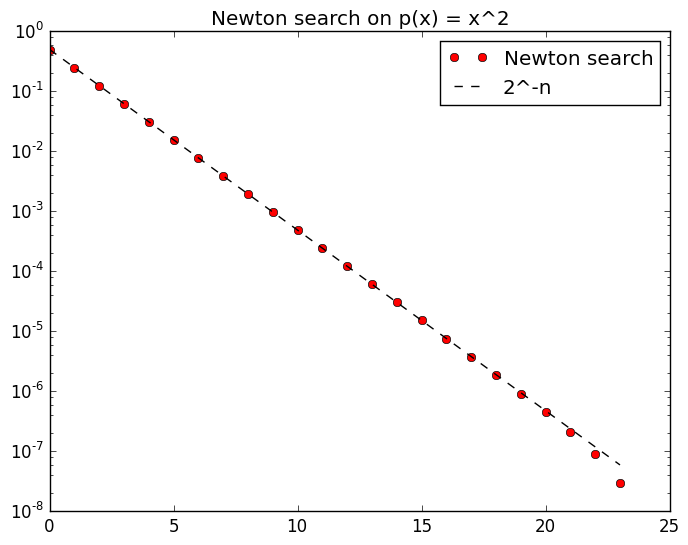Out:
PyObject <matplotlib.text.Text object at 0x7f0028a25290>
In :
# Whoa! Newton search is as slow as bisection on this problem!
# Why? Because the function f(x) = x^2 has slope f'(x) = 2x,
# At the root x=0, the slope is 0. Our derivation of quadratic convergence
# for Newton search required that f' ≠ 0 at the root! It's not hard to show
# that for this function, Newton search will converge at 2⁻ⁿ.


Bonus problem 7. Consider $f(x) = (1-3/(4x))^{1/3}$. What is the root $r$ of this function? What happens when you apply your Newton-method algorithm to this function with a starting guess $x_0 = 1$? Why?

In :
q(x) = (1-3./(4x)).^(1/3)

Out:
q (generic function with 1 method)
In :
# The root of this function is x=3/4. Plug that in and you'll get 0
q(3/4)

Out:
0.0
In :
(r,err) = newtonsearch(q,1,true)

n=0, x=1.0, f(x)=0.6299605249474366
n=1, x=-2.824458311678768e-8, f(x)=298.3380890239169
n=2, x=-1.1297469765312255e-7, f(x)=187.94324181737292
n=3, x=-4.5189156567843757e-7, f(x)=118.39747209054788
n=4, x=-1.8075525275749898e-6, f(x)=74.58596752872286
n=5, x=-7.230194075500259e-6, f(x)=46.986363231992925
n=6, x=-2.8920927194752966e-5, f(x)=29.599787912443215
n=7, x=-0.00011568693804692085, f(x)=18.647243434005137
n=8, x=-0.0004628010532776776, f(x)=11.748387894303308
n=9, x=-0.0018520604862263257, f(x)=7.404443258036132
n=10, x=-0.007421961520331783, f(x)=4.673114243384877
n=11, x=-0.029908186282784827, f(x)=2.9654080850859543
n=12, x=-0.12321073943425324, f(x)=1.9208354760517472
n=13, x=-0.5535664972239525, f(x)=1.330417036011958
n=14, x=-3.4400094485942034, f(x)=1.0679521366183735
n=15, x=-61.09470995115644, f(x)=1.0040753760622745
n=16, x=-15174.380360375248, f(x)=1.0000164748658853
n=17, x=-1.0123653524310206e9, f(x)=1.0000000002469465
n=18, x=-Inf, f(x)=1.0

Out:
(NaN,[NaN,NaN,NaN,NaN,NaN,NaN,NaN,NaN,NaN,NaN,NaN,NaN,NaN,NaN,NaN,NaN,NaN,NaN])
In :
# Wow, Newton search goes totally crazy on this function! Why? Let's plot
#
using PyPlot
x = linspace(0.75001, 5)
plot(x,q(x))
title("q(x) = (1-3/(4x))^(1/3)")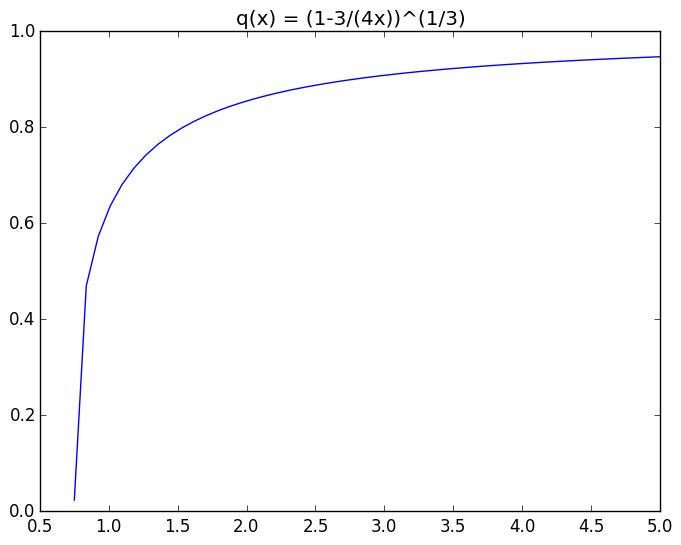Out:
PyObject <matplotlib.text.Text object at 0x7f00288cf450>
In :
# The problem is the slope of this function is infinite at the root!
# q'(x) = 1/3 (1-3/(4x))^(-2/3) (3/4 x^-2), which blows up as x -> 3/4
# Newton search will never be able to find roots with infinite slope